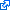# Types of Basis Functions

Although sparse grids are usually constructed with the piecewise linear basis as seen on the page on sparse grid basics, sparse grids can also be employed with other types of hierarchical basis functions to increase the order of approximation or to improve the smoothness of the basis functions. In the following, we present some of these other types.

Note that it is always sufficient to specify the univariate basis functions $\basis{\ell,i}\colon \clint{0, 1} \to \real$ (with level $\ell$ and index $i$), as the multivariate functions $\basis{\*\ell,\*i}\colon \clint{0, 1}^d \to \real$ automatically follow from the tensor product approach.

## Global Polynomials

Global polynomial basis functions are functions $\basis{\ell,i}$ which are one single polynomial on the whole domain $\clint{0, 1}$. The simplest choice are Lagrange polynomials, i.e., polynomials which equal one in $\gp{\ell,i}$ and zero in all other grid points $\gp{\ell,i’}$ ($i’ \not= i$). However, due to Runge’s phenomenon, the standard choice of equidistant grid points lead to large oscillations between the zeros at the grid points, making the basis unstable. Fortunately, sparse grids can also be constructed with different distributions of grid points. For example, if we employ Chebyshev-distributed points to solve the oscillation issues for the global polynomial basis, the resulting sparse grids are called sparse Clenshaw-Curtis grids, a name that stems from Clenshaw-Curtis quadrature.

## Piecewise Polynomials by Bungartz

Another type of basis functions are the piecewise polynomial functions by Bungartz. They are also based on global Lagrange polynomials; however, this time the zeros of $\basis{\ell,i}$ are chosen as the hierarchical ancestors of $\gp{\ell,i}$ up to a specific level, depending on the degree the polynomial is supposed to attain. To solve the issues following from Runge’s phenomenon, the support of $\basis{\ell,i}$ is clipped to $\clint{\gp{\ell,i-1}, \gp{\ell,i+1}}$, where the function is guaranteed to be bounded and non-negative; outside this interval, $\basis{\ell,i}$ is set to zero. This way, the basis functions have the same support as the piecewise linear functions. The advantage of the piecewise polynomials over the piecewise linear basis is the higher order of approximation, which makes them useful for solving specific PDEs.

Literature: 

## B-Splines

Both global polynomials and Bungartz’s piecewise polynomials have their drawbacks: Global polynomials only work for specific grid point distributions, and their global support means that changing a single hierarchical surplus $\surplus{\ell,i}$ changes the resulting linear combinations on the whole domain $\clint{0, 1}$. In contrast, the piecewise polynomials are locally supported, but not continuously differentiable (only continuous), which makes the use in applications problematic, in which continuous gradients are required. An example for such as application is gradient-based optimization, where the direction information from the gradient is used as search direction. If the gradient jumps, this information becomes unreliable.Hierarchical B-splines of degree $p = 3$ with not-a-knot boundary conditions (from , © by Julian Valentin, CC-BY-SA 4.0)

Hierarchical B-splines of degree $p \ge 3$ combine the best of both worlds, as they are locally supported and continuously differentiable up to order $p-1$. In addition, when choosing $p = 1$, we obtain the standard piecewise linear basis as a special case. As splines, B-splines are also piecewise polynomials, but observe specific smoothness conditions for two neighboring polynomial pieces.Properties of B-splines: 1. locality, 2. symmetry, 3. recursion, 4. piecewise polynomial, 5. derivative recursion, 6. unit integral, 7. convolution, 8. generalization of hat/Gaussian functions (from , © by Julian Valentin, CC-BY-SA 4.0)

B-splines have numerous other advantages, for example a recursive structure, nice numerical properties (e.g., the derivative of a B-spline is the weighted difference of two other B-splines), high flexibility, and the higher order of approximation.

Literature: , https://bsplines.org/

 H. Bungartz, “Finite Elements of Higher Order on Sparse Grids,” Habilitation thesis, Technical University Munich, 1998.J. Valentin, “B-Splines for Sparse Grids: Algorithms and Application to Higher-Dimensional Optimization,” PhD thesis, University of Stuttgart, 2019.